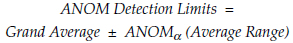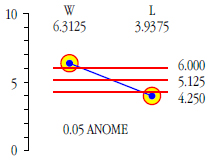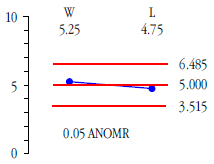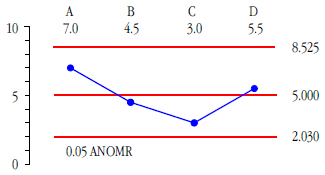#Quality Digest

Featured Product
This Week in Quality Digest Live
Statistics Features
Donald J. Wheeler
What do predictable processes have in common with chaos?
William A. Levinson
People can draw the wrong conclusions due to survivor, survey, and bad news bias.
Donald J. Wheeler
Does your approach do what you need?
Paul Laughlin
Correlation vs. causality
Donald J. Wheeler
In spite of what everyone says to the contrary
Statistics News
New capability delivers deeper productivity insights to help manufacturers meet labor challenges
Day and a half workshop to learn, retain, and transfer GD&T knowledge across an organization
Elsmar Cove is a leading forum for quality and standards compliance
InfinityQS’ quality solutions have helped cold food and beverage manufacturers around the world optimize quality and safety
User friendly graphical user interface makes the R-based statistical engine easily accessible to anyone
Collect measurements, visual defect information, simple Go/No-Go situations from any online device
Good quality is adding an average of 11 percent to organizations’ revenue growth
Ability to subscribe with single-user minimum, floating license, and no long-term commitment
A guide for practitioners and managersBio

Statistics

## Analysis of Means Techniques

### When to use each of the six ANOM techniques

Published: Monday, July 8, 2019 - 12:03

During the past three months James Beagle and I presented columns that made extensive use of analysis of means techniques. Since these techniques may be new to some, this column explains when to use each technique and where to find tables of the appropriate scaling factors.

In 1967, Ellis R. Ott published his analysis of means technique (ANOM) for comparing treatment averages with their grand average. This technique is a generalized version of the average and range chart. However, the assumption that allows this generalization also imposes a restriction of where this technique can be used. The generalization allows us to compute limits with a fixed overall alpha level (the user-specified risk of a false alarm). The restriction is that we can only use ANOM for the one-time analysis of a finite amount data (such as occurs in experimental studies).

Today six related procedures are included under the generic umbrella of ANOM. There is the original analysis of means (ANOM) along with its accompanying analysis of ranges (ANOR). There are two follow-up techniques for making additional comparisons; these are the analysis of main effects (ANOME) and the analysis of mean ranges (ANOMR). Finally there is the analysis of individual values (ANOX), and for m sets of individual values there is the analysis of mean moving ranges (ANOMmR).

### The analysis of means

Assume that you have k treatments or conditions that you wish to compare using some measured characteristic. Also assume that you have n measurements collected under each of these k treatments where n is two or greater. Here you can compute an average and a range for each of the k treatments. The k averages may be compared using an ANOM chart.

The k treatment averages are plotted as a running record. The grand average of all k treatments is used as the central line. And the detection limits are computed using the average of the k treatment ranges.Where ANOMα is the scaling factor for k treatments of size n that will result in an overall risk of a false alarm of alpha. Tables of ANOM scaling factors may be found in sources  and  in the list at the end of this article.

Since data from an experimental study are rarely organized in time-order, run tests are inappropriate for an ANOM chart. The only type of signal to consider here is a point outside the limits.

### The analysis of ranges

The k treatment ranges used above can be checked for homogeneity using an analysis of ranges (ANOR) chart. The k treatment ranges are plotted in the same order as the k treatment averages in the ANOM chart above, and the average range is used as the central line. The upper ANOR detection limit is found using:Where the ANORα scaling factor depends upon n, k, and alpha. Tables for ANOR may be found in sources  and  in the list at the end of this article.

A combined ANOM and ANOR chart is shown in figure 1. Since it looks like an average and range chart I always recommend labeling each chart as being ANOM or ANOR along with what overall alpha level was used. Here we have k = 8 treatments with n = 4 observations collected for each treatment. The grand average is 5.125 and the average range is 5.000. With n = 4 and k = 8, the 5-percent ANOM scaling factor is 0.668, while the 5-percent ANOR scaling factor is 2.138.Figure 1: Combined ANOM and ANOR charts

Here we find two averages that are detectably different from the grand average (with p-value less than 0.05), and no ranges that indicate excessive variation within any treatment.

### Fully crossed studies

When an experimental study involves more than one factor, each “treatment” will consist of some combination of factor levels. For example in the ANOM chart of figure 1 the eight treatments represent combinations of two different factors. One factor was a type of heat treatment. The second factor was the machine used to process the parts following their heat treatment. With four machines and two levels of heat treatment the eight treatments above represent all possible machine by heat treatment combinations. When all possible factor level combinations are present the experiment is said to be fully crossed. With a fully-crossed study we can use ANOM to see if any of the treatments differ from the overall average and then we can use the analysis of main effects (ANOME) to compare the levels for each of the factors in the experiment.Figure 2: ANOM chart showing the factor levels for each treatment

### The analysis of main effects

ANOME (a-nom-e) allows us to ask which levels of each factor are detectably different from the grand average. For figure 2 we begin by computing an average for each level of heat treatment. For heat treatment W this average is 6.3125. For heat treatment L this average is 3.9375. To find the appropriate detection limits we use the original grand average of 5.125 and the original average range of 5.000 along with the ANOME scaling factor. Tables of these scaling factors may be found in sources , , and  in the list at the end of this article. Here the scaling factor will depend upon the original n = 4, and k = 8, plus the number of levels being compared, m = 2, and the overall alpha level for the chart. With a 5-percent risk of a false alarm, this ANOME scaling factor is 0.175. The formula for the detection limits is:Figure 3: ANOME for heat treatment W vs. L

The main effect ANOM for heat treatments is shown in figure 3. The two heat treatments are detectably different, and our estimate of this difference is that heat treatment W results in responses that are about 2.4 units greater than we find with heat treatment L.

To compare machines we compute an average for each machine. With n = 4, k = 8, alpha = 0.05, and m now equal to 4 we find the ANOME scaling factor to be 0.391, resulting in the ANOME chart in figure 4.Figure 4: ANOME for machines A, B, C, and D

While machines A and B are not detectably different from the grand average, machines C and D are detectably different. Machine C is 3.25 units below the grand average while machine D is 2.75 units higher than the grand average.

### The analysis of mean ranges

The ANOMR (a-nom-r) allows us to compare the average ranges for the levels of each factor in a fully-crossed study. This allows us to determine if certain levels of a factor result in more or less variation in the response variable. (When the ANOR chart in figure 1 shows no evidence of treatments with increased levels of variation the ANOMR may not be of interest.) Nevertheless, we shall compare the variation found within each level of heat treatment and within each machine.

Tables of the ANOMR scaling factors may be found in sources  and  in the list at the end of this article. To compare mean ranges for the two levels of heat treatment we begin by computing the average range for each level. For heat treatment W the average range is 5.25, while for heat treatment L the average range is 4.75. Since ANOMR limits are not always symmetric about the original average range (5.00) we use two scaling factors. With n = 4, k = 8, and comparing m = 2 average ranges using an alpha level of 5 percent, the two scaling factors are UMR = 1.297 and LMR = 0.703.The detection limits are computed using the original overall average range of 5.000 from figure 1. These limits are 6.485 and 3.515. The ANOMR chart in figure 5 shows no evidence of different amounts of variation within either level of heat treatment.Figure 5: ANOMR for heat treatment W vs. L

For comparing the variation within the m = 4 machines we use UMR = 1.705 and LMR = 0.406, resulting in limits of 8.525 and 2.030.Figure 6: ANOMR for machines A, B, C, and D

Since none of the average ranges in figure 6 falls outside the detection limits we have no evidence of any differences in the variation for the parts produced on the different machines.

The four ANOM techniques listed above are all for use with experimental studies where there are k treatments with n observations obtained for each treatment. The following ANOM techniques are intended for data sets with a different structure.

### The analysis of individual values

ANOX (a-nox) is a technique to check for signals where there should be no signals. It examines a set of observations that should all be the same to see if there are any exceptional values present.

Given a set of k individual values and given the k–1 successive differences between these values (i.e., k–1 moving ranges), the homogeneity of this set of k individual values may be examined by computing ANOX detection limits:Where the scaling factor ANOXα depends upon the desired alpha level for the test and k = the number of individual values being tested. Tables for the ANOX scaling factors are given in source  in the list below.

When the k values are actually homogeneous these limits will bracket all of the individual values 100[1–alpha] percent of the time, and the smallest, or largest, of the k individual values can be expected to fall outside these limits only 100[alpha] percent of the time.

If a single individual value falls outside the ANOX limits then you either have a rare event with probability alpha, or you have a nonhomogeneous set of individual values. Of course, as more values fall outside the ANOX limits, and as they fall further outside the ANOX limits, the evidence of nonhomogeneity becomes stronger.

In applying this test for homogeneity the individual values must not be arranged in a ranking (where the values are arranged in either an ascending or descending numerical order). Such orderings undermine the method of successive differences, which is the foundation of both the XmR chart and ANOX. (This caveat also applies to the XmR charts used in ANOMmR.)

It is always best to use the data in the order in which they naturally occur. If time-order information is available, this ordering is preferred. If the time-order information has been lost, use the order in which the data are presented (as long as it is not a ranking).

In most one-time tests we are trying to establish that a signal exists. In a test of homogeneity we are trying to establish that a signal does not exist. This difference will affect the way we use a test for homogeneity.

When we use a 5-percent ANOX or a 10-percent ANOX and find no evidence of a lack of homogeneity, then we can be comfortable with the conclusion that the data are probably homogeneous. We will have obtained a fairly strong result.

When we use a 1-percent ANOX and find evidence of a lack of homogeneity, then we can be comfortable with the conclusion that the data are nonhomogeneous.

Since the skeptic will assume the data are not homogeneous until proven otherwise, and since the strong conclusion regarding homogeneity requires a larger alpha level, we should be careful about using a 1-percent ANOX to conclude that the data are homogeneous.

In addition, it is important to remember that with ANOX the alpha level is the probability that either the maximum or minimum of the points will fall outside the limits.

So if we perform a 10-percent ANOX with k = 100 points, the alpha level of 10 percent does not mean that we expect 10 out of 100 values to fall outside the limits. Instead, it means that there is a 10-percent chance that either the smallest or the largest of the 100 values might fall just outside the limits. When a false alarm occurs with ANOX it will tend to show a single point just outside the limit, rather than having a point noticeably outside the limits. The judgment that a data set is not homogeneous can depend upon how far outside the limits a point may fall as well as how many points are outside the limits.

Examples of the use of ANOX may be found in source  in the list below.

### The analysis of mean moving ranges

ANOMmR (a-nom-m-r) compares m average moving ranges to discover if there are detectable differences in variation present in a set of m different sets of k individual values. This means that each average moving range is based upon (k–1) two-point moving ranges. That is, each average moving range comes from an XmR chart that has a baseline of k original data.

The central line of the ANOMmR chart is the grand average of the m average moving ranges. The upper and lower detection limits of an ANOMmR chart are found by multiplying the grand average moving range by scaling factors labeled as LL and UL in the tables. These ANOMmR scaling factors will depend upon three quantities: the alpha level; m = number of average moving ranges being compared; and k = the number of original X values in each of the XmR charts.Tables of these scaling factors and an example of the ANOMmR chart are given in source .

### Summary

Do not be fooled by the simplicity of these ANOM techniques. While the computations are intuitive and easy, these techniques have been found to be essentially as sensitive (powerful) as other, more complex, traditional techniques. Moreover, the clarity provided by graphs makes the ANOM techniques much easier to interpret and to use in communicating discoveries to others.

While the first four ANOM techniques listed below are designed to help you find signals contained within experimental data, the last two ANOM techniques are essentially tests for homogeneity.Figure 7: Six ANOM techniques

The formulas given here, and the scaling factors given in the tables in the sources below are based on the use of either the within-subgroup ranges or the moving ranges for individual values. Why not use the within-subgroup standard deviations? First, the use of within-subgroup ranges is in keeping with Ellis Ott’s original intention of providing a user-friendly technique. Second, until the subgroup size exceeds 15, the range is essentially as efficient as the standard deviation statistic, and is much easier for everyone to understand. For more on this see “Why Use Ranges?” (Quality Digest Daily, Feb. 3, 2014). The two-point moving ranges are used with sequences of individual values because they are 100-percent efficient and are more robust than other measures of dispersion. When simplicity, efficiency, and clarity combine, why go anywhere else?

### Sources for tables ANOM technique scaling factors

1. Donald J. Wheeler, “The Analysis of Experimental Data,” (Quality Digest Daily, Jan. 6, 2014). This article has ANOM, ANOR, and ANOME scaling factors for alpha levels of 10%, 5%, and 1%; for subgroup sizes of n = 2 to 10; and for k = 2 to 12 treatments.

2. James Beagle III and Donald J. Wheeler, “When Are Instruments Equivalent? Part Three,” (Quality Digest, June 10, 2019). This article has ANOME and ANOMR scaling factors for an alpha level of 5%; for subgroups of size n = 2 to 5; and for k = 2 to 24 treatments.

3. Donald J. Wheeler, Analyzing Experimental Data, (SPC Press, 2013). This book has ANOM, ANOR, ANOME, and ANOMR scaling factors for alpha levels of 10%, 5%, and 1%; for subgroup sizes of n = 2 to 20; and for k = 2 to 60 treatments.

4. Donald J. Wheeler and James Beagle III, “ANOX: The Analysis of Individual Values,” (Quality Digest, Sept. 4, 2017). This article has ANOX scaling factors for alpha levels of 10%, 5%, and 1% for examining a sequence of k = 8 to 480 individual values for homogeneity.

5. Donald J. Wheeler and James Beagle III, “When Are Instruments Equivalent? Part Two,” (Quality Digest, May 6, 2019). This article has ANOMmR scaling factors for alpha levels of 10%, 5%, and 1%; for comparing up to m = 20 average moving ranges where each average moving range comes from an XmR chart based on k = 5 to 50 original values.

### Discuss